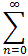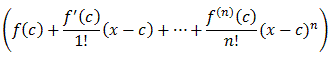#Interactive Real Analysis

Next | Previous | Glossary | Map

## 8.4. Taylor Series

### Proposition 8.4.10: The Geometric Series

1/1-x = 1 + x + x2 + x3 + x4 + ... =xn for -1 < x < 1

For the geometric series we can actually compute the remainder in Taylor's theorem exactly. Recall that we have previously shown that if we define Sn(x) = 1 + x + x2 + ... + xn we can find a closed form for Sn(x) by multiplying Sn(x) by x and then subtracting Sn(x) - x Sn(x) to get:

Sn(x) = 1-xn+1/1-x

or equivalently

1 + x + x2 + ... + xn = 1 - xn+1/1 - x = 1/1-x - xn+1/1-x

We can rearrange the terms to get:

1/1-x - (1 + x + x2 + ... + xn) = xn+1/1-x

But this means that we have (with c = 0)

|f(x) -| = |Rn+1(x)| = | xn+1 / 1 - x |

Thus, we have found the remainder exactly! And since |x| < 1 it is now clear that this remainder converges to zero, so that by Taylor's theorem the Taylor series converges to the function, as required.Next | Previous | Glossary | Map